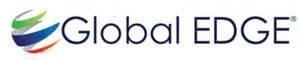1.If the number 481 * 673 is completely divisible by 9, then the smallest whole number in place of * will be:
A.2
B.5
C.6
D.7
E.None of these
Ex:Sum of digits = (4 + 8 + 1 + x + 6 + 7 + 3) = (29 + x), which must be divisible by 9.
Therefore, x = 7.

2.3897 x 999 = ?
A.3883203
B.3893103
C.3639403
D.3791203
E.None of these
EX:3897 x 999 = 3897 x (1000 – 1)

= 3897 x 1000 – 3897 x 1

= 3897000 – 3897

= 3893103.

3.Sakshi can do a piece of work in 20 days. Tanya is 25% more efficient than Sakshi. The number of days taken by Tanya to do the same
piece of work is:
A.15
B.16
C.18
D.25
Ex:Ratio of times taken by Sakshi and Tanya = 125:100 = 5:4.
Suppose Tanya takes x days to do the work.
5 : 4 :: 20 : x ⇒ x =(4 x 20)/5
⇒ x = 16 days
Hence, Tanya takes 16 days to complete the work.

4.A and B together can do a piece of work in 30 days. A having worked for 16 days, B finishes the remaining work alone in 44 days. In
how many days shall B finish the whole work alone?
A.30 days
B.40 days
C.60 days
D.70 days
Ex:Let A’s 1 day’s work = x and B’s 1 day’s work = y.
Then, x + y =1/30 and 16x + 44y = 1.
Solving these two equations, we get: x =1/60 and y =1/60
Hence, B alone shall finish the whole work in 60 days.

5.A train left station at A hour B minutes. It reached station Y at B hour C minutes on the same day, after travelling C hours A
minutes (clock shows time from 0 hours to 24 hours). Number of possible value of A is?
A.0
B.1
C.2
D.3
Ex:A hours + C ours = B hours …..(i)
A, C and B cannot have values greater than or equal to 24
B minutes + A minutes = C minutes …..(ii)
Looking at two equation, we get no value of A satisfies both equation.

6.An aeroplane covers a certain distance at a speed of 240 kmph in 5 hours. To cover the same distance in 5/3 hours, it must travel
at a speed of:
A.300 kmph
B.360 kmph
C.600 kmph
D.720 kmph
Ex:Distance = (240 x 5) = 1200 km.
Speed = Distance/Time
Speed =1200/(5/3)km/hr
∴ Required speed = 1200 x (3/5)km/hr = 720 km/hr

7.A merchant marks his goods up by 75% above his cost price. What is the maximum % discount that he can offer so that he ends up
selling at no profit or loss?
A.75%
B.46.67%
C.300%
D.42.85%
Ex:Let us assume that the cost price of the article = Rs.100
Therefore, the merchant would have marked it to Rs.100 + 75% of Rs.100 = 100 + 75 = 175.
Now, if he sells it at no profit or loss, he sells it at the cost price.i.e. he offers a discount of Rs.75 on his selling price of
Rs.175
Therefore, his % discount = (75/175) x 100= 42.85%

8.The average weight of a class of 24 students is 36 years. When the weight of the teacher is also included, the average weight
increases by 1kg. What is the weight of the teacher?
A.60 kgs
B.61 kgs
C.37 kgs
D.None of these
Ex:The average weight of a class of 24 students = 36 kgs.
Therefore, the total weight of the class = 24 x 36 = 864 kgs
When the weight of the teacher is included, there are 25 individuals.
The average weight increases by 1kg. That is the new average weight = 37 kgs.
Therefore, the total weight of the 24 students plus the teacher = 25 x 37 = 925
Weight of the teacher = Weight of 24 students + teacher – weight of 24 students = 925 – 864 = 61 kgs

9.Ayesha’s father was 38 years of age when she was born while her mother was 36 years old when her brother four year younger to her
was born. What is the difference between the ages of her parents?
A.2 years
B.4 years
C.6 years
D.8 years
Ex:Mother’s age when ayesha’s brother was born = 36 years.
Father’s age when Ayesha’s brother was born = (38 + 4) = 42 years.
So, required difference = ( 42 – 36 ) years = 6 years.

10.Two number are in the ratio 3 : 5. If 9 is subtracted from each, the new numbers are in the ratio 12 : 23. The smaller number is:
A.27
B.33
C.49
D.55
Ex:Let the numbers be 3x and 5x.
Then, (3x – 9)/5x – 9=12/23
⇒ 23(3x – 9) = 12(5x – 9)
⇒ 9x = 99
⇒ x = 11.
∴ The smaller number = (3 x 11) = 33.